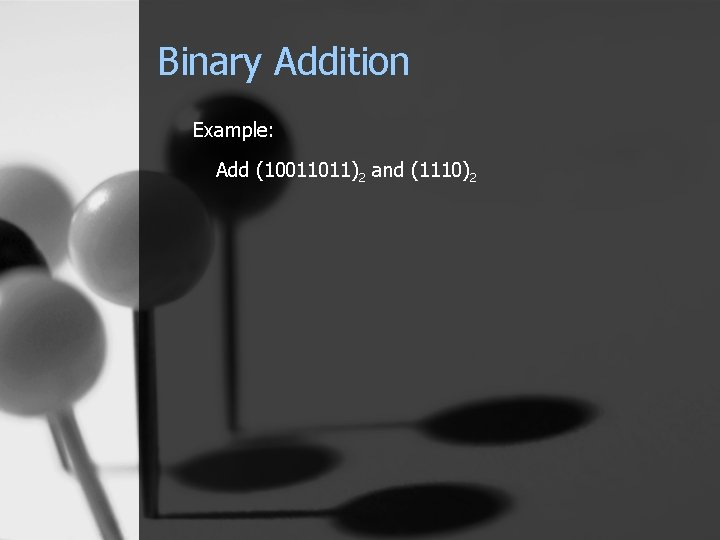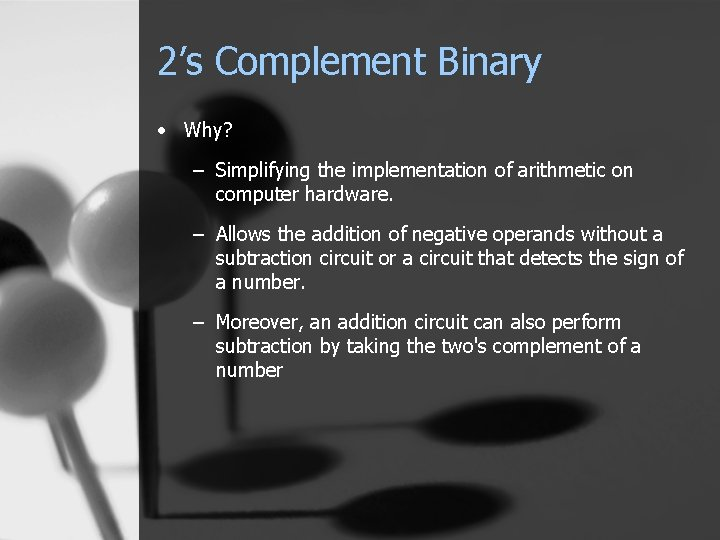# Number Systems Today Decimal Hexadecimal Binary Unsigned Binary

• Slides: 13Number Systems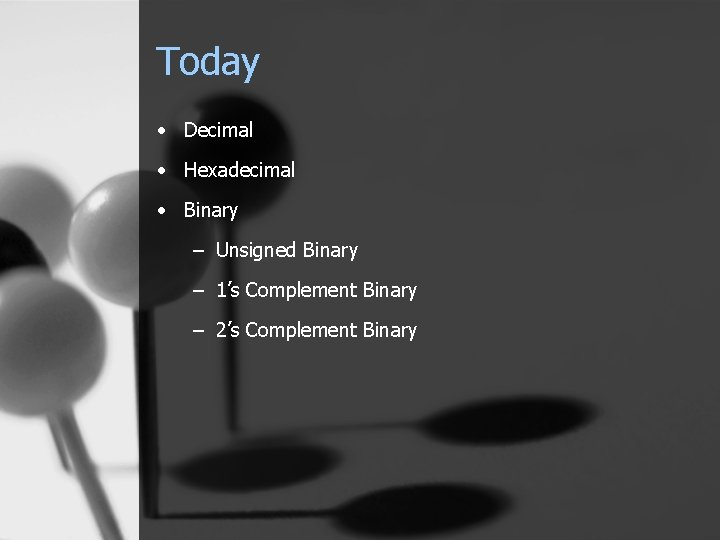Today • Decimal • Hexadecimal • Binary – Unsigned Binary – 1’s Complement Binary – 2’s Complement BinaryDecimal (base 10) ( 0, 1, 2, 3, 4, 5, 6, 7, 8, 9 ) Binary (base 2) ( 0, 1 ) Hexadecimal (base 16) ( 0, 1, 2, 3, 4, 5, 6, 7, 8, 9, A, B, C, D, E, F )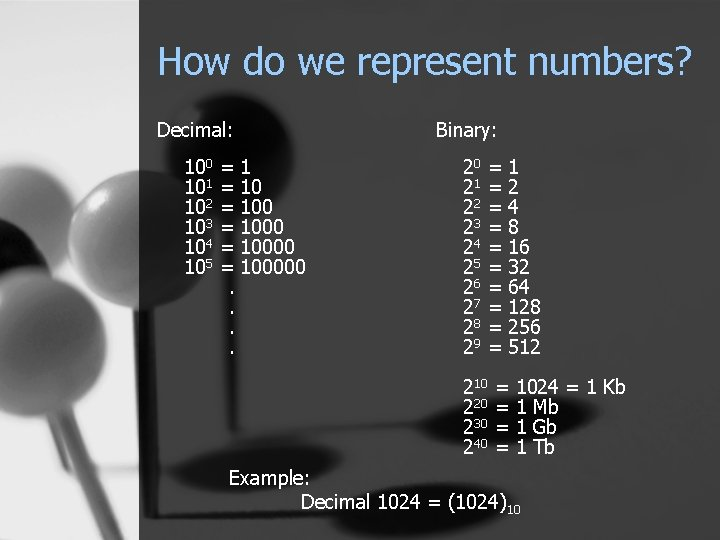How do we represent numbers? Decimal: Binary: 100 101 102 103 104 105 20 21 22 23 24 25 26 27 28 29 =1 = 10000 = 100000. . = = = = = 210 220 230 240 1 2 4 8 16 32 64 128 256 512 = = 1024 = 1 Kb 1 Mb 1 Gb 1 Tb Example: Decimal 1024 = (1024)10Notes: Counting Chart ( 99 )10 + 1 = ? ? ( FF )16 + 1 = ? ? ( 100 )10 ( 100 )16Conversion • Decimal (Base 10) --> Binary (Base 2) Step 1 - Divide the Number by 2 Step 2 - If the result has a remainder, --> add 1 as the current MSB Otherwise --> add 0 as the current MSB Step 3 - Finish when result < base --> Add Quotient as the final MSB Example: Convert (1000)10 to Binary (base 2)Conversion • Decimal (Base 10) -> Hexadecimal (Base 16) Step 1 - Divide the Number by 16 Step 2 - Take the remainder as the current MSB Step 3 - Finish when result < base --> Add Quotient as the final MSB Example: Convert (1000)10 to Hexadecimal (base 16)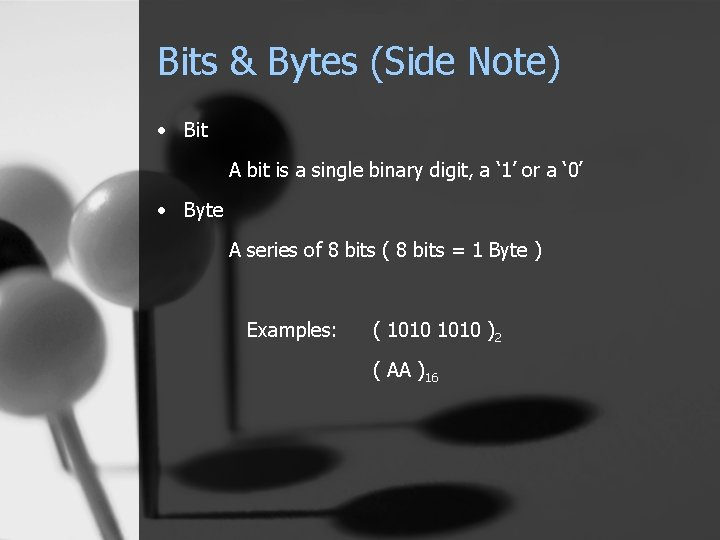Bits & Bytes (Side Note) • Bit A bit is a single binary digit, a ‘ 1’ or a ‘ 0’ • Byte A series of 8 bits ( 8 bits = 1 Byte ) Examples: ( 1010 )2 ( AA )16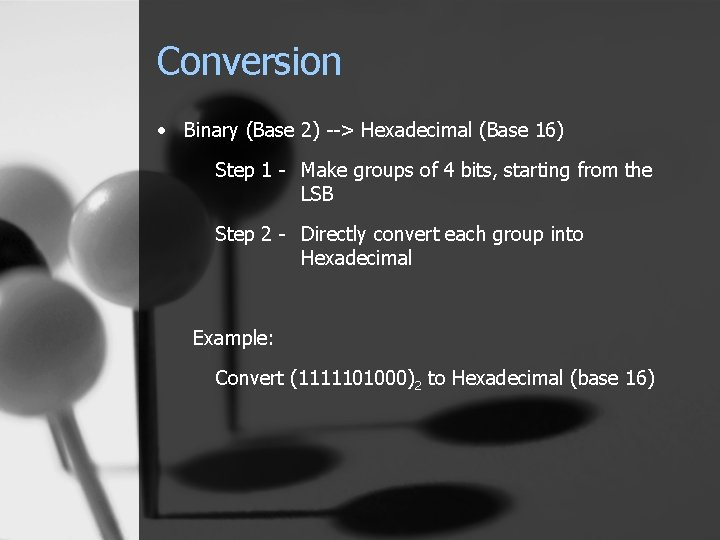Conversion • Binary (Base 2) --> Hexadecimal (Base 16) Step 1 - Make groups of 4 bits, starting from the LSB Step 2 - Directly convert each group into Hexadecimal Example: Convert (1111101000)2 to Hexadecimal (base 16)